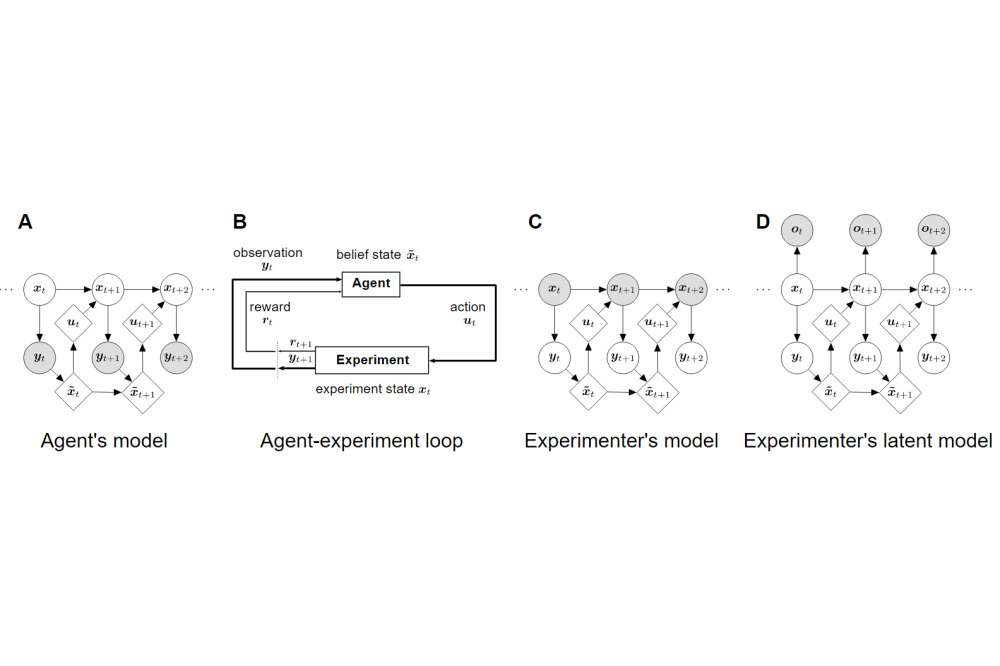# Inverse Optimal Control Adapted to the Noise Characteristics of the Human Sensorimotor SystemStraub, Dominik_Inverse Optimal Control Adapted to the Noise Characteristics of the Human Sensorimotor System

Figure 1: The agent-experimenter loop and its formalization as POMDP together with the inference problems from the agent’s point of view and from the experimenter’s point of view. This conceptual framework is the basis of our inverse optimal control method.

Dominik Straub

## Introduction

Normative computational models of human sensorimotor behavior based on optimal feedback control with signal-dependent noise have been able to account for many phenomena including online corrections, redundancy, synergies, and uncontrolled manifolds of movements. In past research, a cost function needed to be assumed for the respective task, and agreement between the predictions of optimal behavior by the model and empirical movement trajectories had to be assessed. In this project, we develop an algorithm for inverse optimal control with signal-dependent noise that allows inferring the cost function underlying behavior from observed trajectories. Estimating the cost function based on recorded behavior can provide insights that help to understand how humans act in their daily environment.

## Methods

In order to build the intended algorithm, we derived a tractable approximation for the likelihood of observed trajectories in linear-quadratic systems based on a variational formulation. Specifically, the non-Gaussian signal-dependent noises were approximated with Gaussian distributions, allowing for a closed-form approximation of the likelihood function in the inverse optimal control problem. Using existing gradient-free optimization methods, this formulation enables the search for the parameters which maximize this approximate likelihood and are therefore most likely to have produced the data. In each step of the optimizer, an optimal filtering and control problem needs to be solved. We implemented an algorithm for simulating trajectories, our proposed inverse optimal control method, as well as functionality to evaluate the results. Finally, we systematically evaluated our method on a variety of different problems and parameter ranges.

## Results

In the past project period, we were able to develop the intended inverse optimal control method and evaluated it on a wide range of different optimal control problems, i.e., a reaching task, saccadic eye movement task, and randomly-generated problems. We were able to show that it can be used in all tasks to recover the underlying cost function of generated noisy trajectories in linear-quadratic systems with signal-dependent noises. Trajectories simulated using the inferred parameters were shown to be reasonably close to the original data.

## Discussion

The developed method can be used to estimate underlying costs in animal and human sensorimotor data. By recovering the cost function underlying observed behavior, we can get insights to human and animal planning and decision-making strategies. A limitation of the approach is that in its current form it is restricted to problems with linear dynamics and quadratic cost functions. In the future, we aim to extend this method further to non-linear problems and want to use it to explain variability in real data. To achieve this, there are several different approaches, which could be viable. On the one hand, there are extensions of the optimal control methods, which our inverse optimal control method relies on, to non-linear problems using iterative linearization methods. On the other hand, the recent successes of deep neural networks in reinforcement-learning domains suggest the use of such architectures to approximate optimal control. In our future work, we would like to investigate the feasability of using neural networks within our inverse optimal control framework.

## Last Update

• Last Update: 2022-06-08 12:49